The best tools to make your project dreams come true

﻿

# Intro to TinyML Part 1: Training a Model for Arduino in TensorFlow

## By ShawnHymel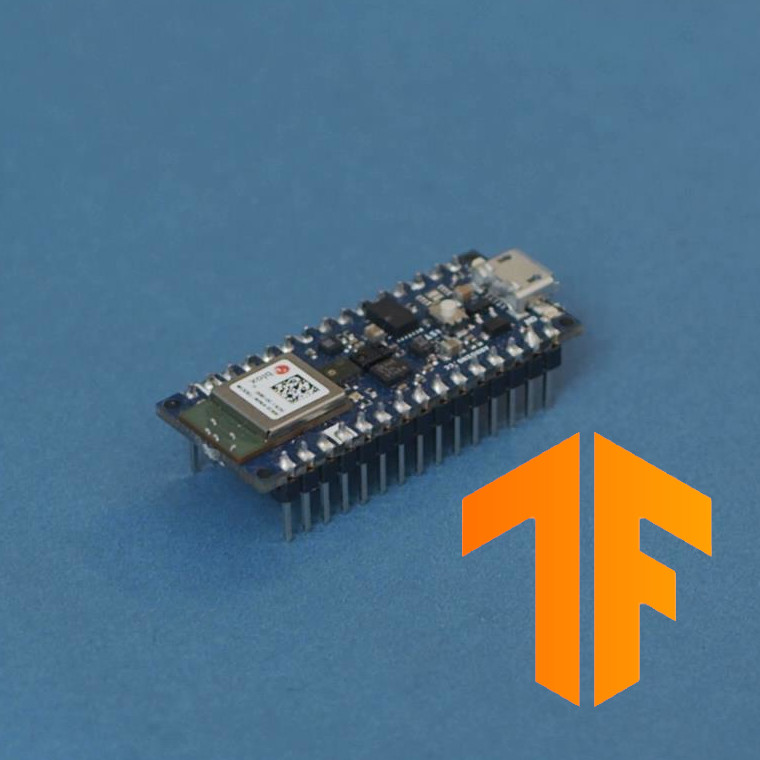When most of us think about artificial intelligence (AI) and machine learning (ML), we usually conjure up thoughts about home assistants, autonomous robots, and self-driving cars. However, the world of ML encompasses much more than that. Any time we train a mathematical model to help a computer make predictions, decisions, or classifications without being explicitly told to do so, we are using ML.

In most cases, useful machine learning algorithms require copious amounts of computing resources (CPU cycles and RAM). However, TensorFlow Lite has recently released an experimental version that runs on several microcontrollers. Assuming we can create a model that fits on a device with limited resources, we can start turning embedded systems into tiny machine learning (TinyML) devices.

In this tutorial, we will create a neural network that is capable of predicting the output of the sine function. We will then convert this model to a TensorFlow Lite (TFLite) model and examine it using Netron.

If you would like to see an explanation of these steps in video form, check out this YouTube video:

Model Description

We are going to create a 3-layer, fully connected neural network that predicts the output of the sine function. As such, we are using it as a regression model.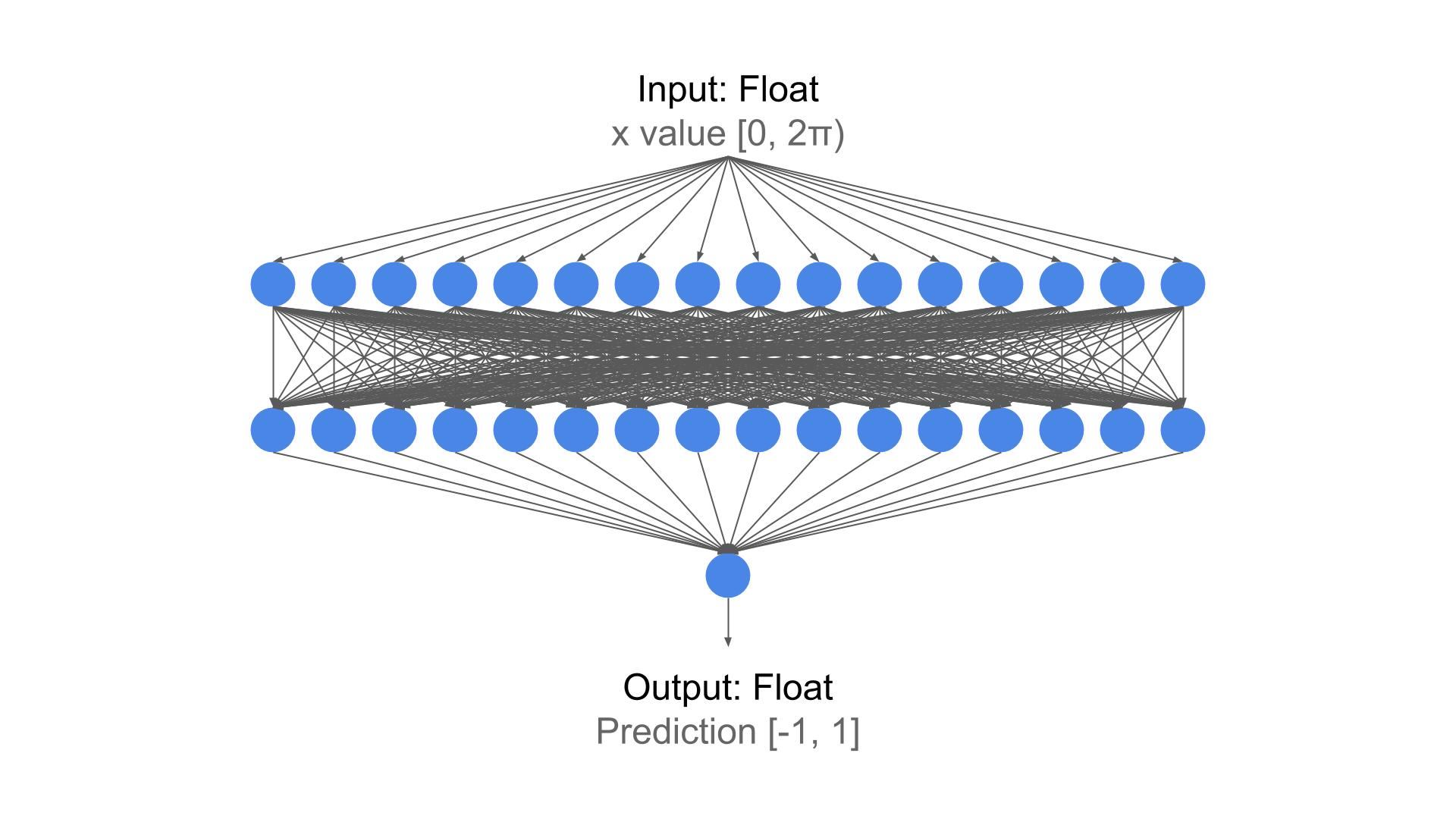The idea is to train a model that accepts values between 0 and 2π and then outputs a value between -1 and 1. If we label the input value as x and the output as y, then the model should be able to predict y = sin(x).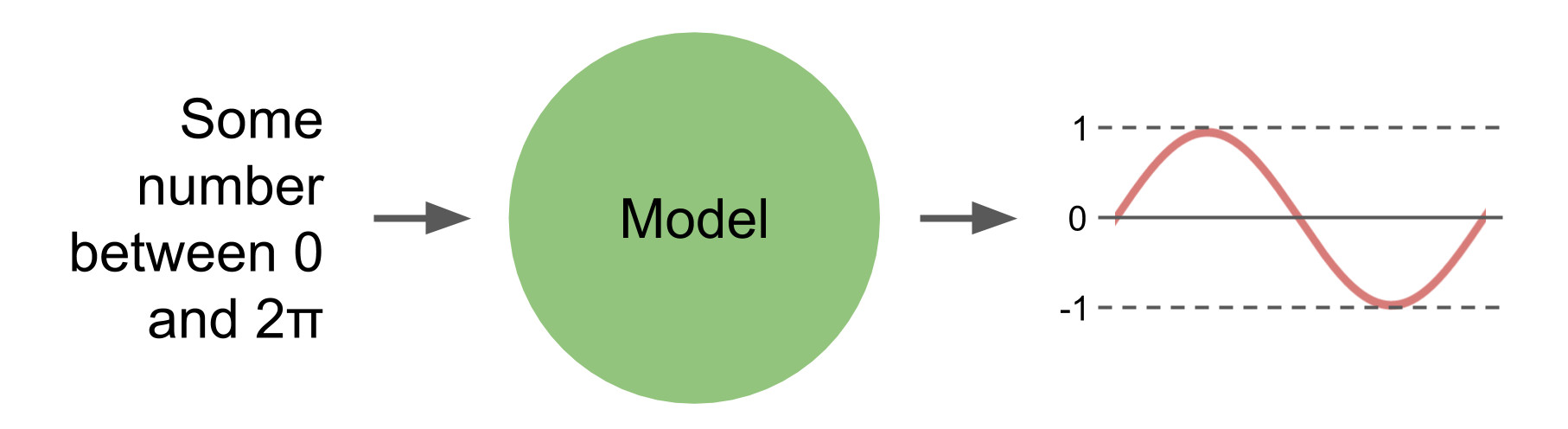This might be one of the most inefficient, most roundabout ways to calculate a sinewave. However, it allows us to play with a small neural network with some nonlinearity and load it onto a microcontroller.

TensorFlow includes a converter class that allows us to convert a Keras model to a TensorFlow Lite model. The TensorFlow Lite model is stored as a FlatBuffer, which is useful for reading large chunks of data one piece at a time (rather than having to load everything into RAM).

Once we have created and trained the model, we will run the TensorFlow Lite converter to create a tflite model. From there, we will need to store the tflite model as a series of bytes in a C constant array (either in a .c or .h file). This will allow us to load the model and use it for inference with the TensorFlow Lite for Microcontrollers library.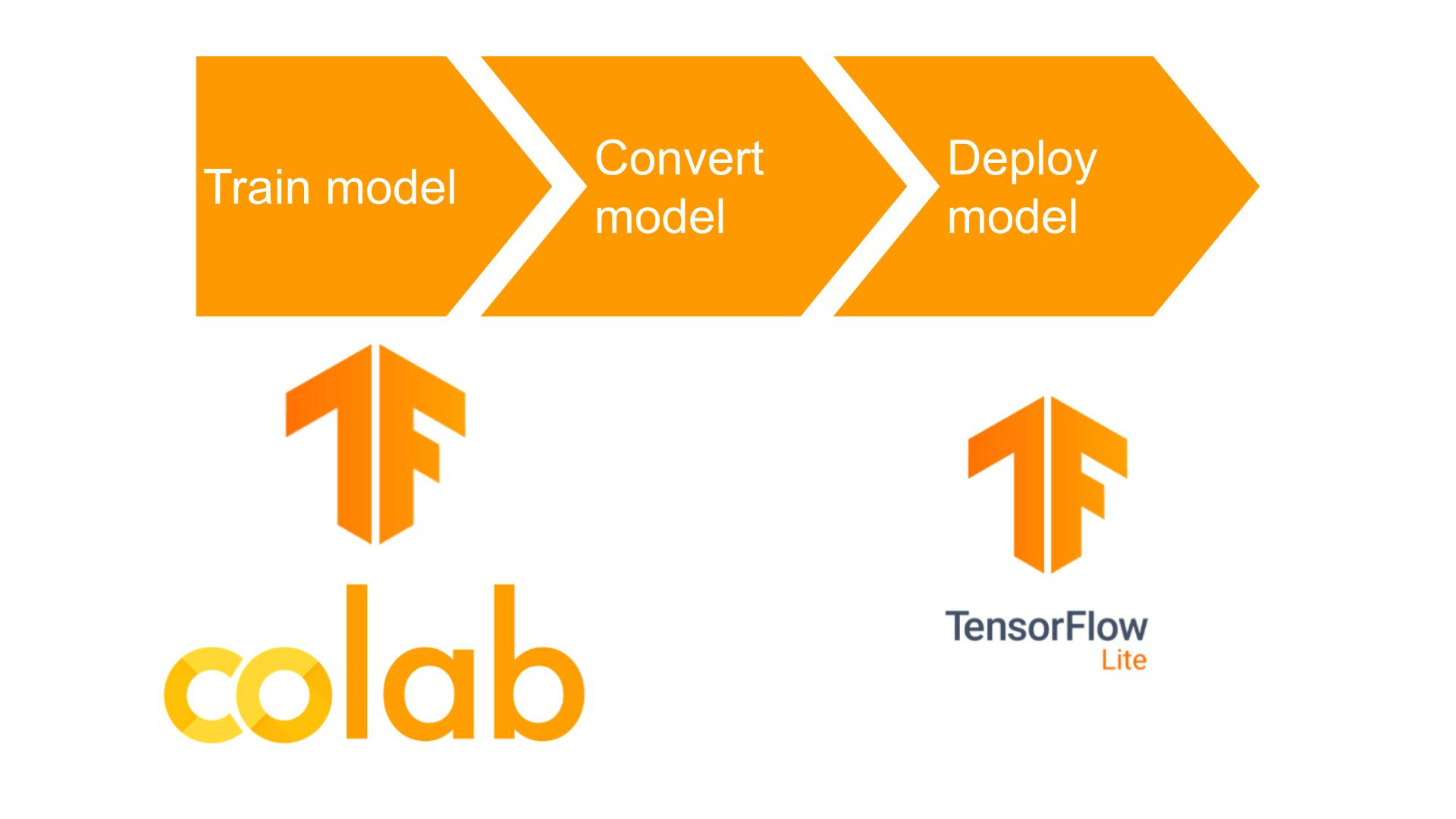TensorFlow Lite has support for a few microcontroller boards, which you can see the list here. At the time this tutorial was released, only 8 microcontroller boards were supported. While there is an arduino library available for TensorFlow Lite, only the Nano 33 BLE Sense was supported. As a result, we will be using the Nano 33 BLE Sense in the second part of this tutorial series.

Google Colab is a web-based Jupyter Notebook interface that runs on a Linux virtual machine (VM). It comes pre-loaded with most of the popular machine learning packages, and it's free!

While you can play around with various machine learning algorithms in Colab, you’re limited in VM resources and time. If you don’t engage with the interface for 90 min, you will be disconnected, and every 12 hours, the runtime will reset.

If you wish to train models for longer or need more resources, you will need to pay for the Pro version or set up your own machine learning development machine (local or server).

Note that most of the code below should also run locally in Python or on Jupyter Notebook. If you wish to run TensorFlow locally, please see this tutorial.

Model Training

Please note that the original code is based on Pete Warden’s work for TensorFlow Lite. I have tweaked the example some to work better on video.

Head to Colab and click New Notebook. Give that notebook a unique name, like “tflite-sinewave-training.ipynb.”

The code is best viewed in Notebook form so you can see example outputs. I will discuss some of the important pieces of code in this tutorial, but if you’re interested in running the Notebook yourself and dissecting the code, please see this Gist: https://gist.github.com/ShawnHymel/79237fe6aee5a3653c497d879f746c0c

At the top, we select the version of TensorFlow we wish to use. While I originally specified “2.1,” this magic command only deals with major versions. As a result, it’s recommended to use:

Copy Code
`%tensorflow_version 2.x`

After that, we import our necessary packages and print their versions, which can be helpful when asking for help on various forums, as people will usually request to see which version of things you’re using.

We then specify various settings, including the number of samples we wish to use and the percentage of samples we wish to set aside for validation and test sets.

From there, we generate a random sampling of x values to be used to train our model:

Copy Code
`# Generate some random samplesnp.random.seed(1234)x_values = np.random.uniform(low=0, high=(2 * math.pi), size=nsamples)plt.plot(x_values)`

We then calculate sin(x) for each sample and add a little bit of random, Gaussian noise to the output. This helps us ensure the model is an estimation and not a precise representation of a sinewave.

Copy Code
`# Create a noisy sinewave with these valuesy_values = np.sin(x_values) + (0.1 * np.random.randn(x_values.shape))plt.plot(x_values, y_values, '.')`

Next, we split the data up into training, validation, and test sets. It’s usually a good idea to view (or plot) your input data and labels, if possible. Because input and output are only one value here (as opposed to a many-dimensional array), we can visualize the relationship between inputs and outputs more easily:

Copy Code
`# Split the dataset into training, validation, and test setsval_split = int(val_ratio * nsamples)test_split = int(val_split + (test_ratio * nsamples))x_val, x_test, x_train = np.split(x_values, [val_split, test_split])y_val, y_test, y_train = np.split(y_values, [val_split, test_split])# Check that our splits add up correctlyassert(x_train.size + x_val.size + x_test.size) == nsamples# Plot the data in each partition in different colors:plt.plot(x_train, y_train, 'b.', label="Train")plt.plot(x_test, y_test, 'r.', label="Test")plt.plot(x_val, y_val, 'y.', label="Validate")plt.legend()plt.show()`

This should give us a plot as shown here:Now that we have our data prepared, it’s time to create the model. We’ll use a 3-layer, fully-connected (dense) neural network.

Copy Code
`# Create a modelmodel = tf.keras.Sequential()model.add(layers.Dense(16, activation='relu', input_shape=(1,)))model.add(layers.Dense(16, activation='relu'))model.add(layers.Dense(1))`

Then, we can compile it with our set optimizers and loss functions, followed by the actual training procedure:

Copy Code
`# Add optimizer, loss function, and metrics to model and compile itmodel.compile(optimizer='rmsprop', loss='mae', metrics=['mae'])`

Copy Code
`# Train modelhistory = model.fit(x_train,                    y_train,                    epochs=500,                    batch_size=100,                    validation_data=(x_val, y_val))`

After a few minutes, you should have a fully trained model. We can plot the model’s predicted sine function versus the test data to ensure that we have something that approximates a sinewave:

Copy Code
`# Plot the training historyloss = history.history['loss']val_loss = history.history['val_loss']epochs = range(1, len(loss) + 1)plt.plot(epochs, loss, 'bo', label='Training loss')plt.plot(epochs, val_loss, 'b', label='Validation loss')plt.title('Training and validation loss')plt.legend()plt.show()`

The output of this code should give us a plot that looks like the following: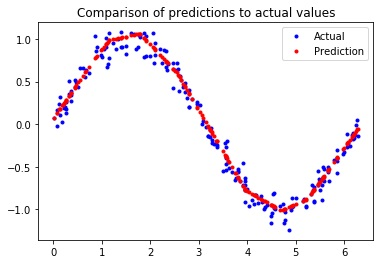As you can see, our model should give us something that approximates a sinewave, even if it’s not exactly correct.

Convert to TensorFlow Lite Model

Now that we have a Keras model that has been trained, we need to convert it to something that our microcontroller can use. TensorFlow has a built-in converter function that will save the model as a TensorFlow Lite model file (stored as a FlatBuffer):

Copy Code
`# Convert Keras model to a tflite modelconverter = tf.lite.TFLiteConverter.from_keras_model(model)converter.optimizations = [tf.lite.Optimize.OPTIMIZE_FOR_SIZE]tflite_model = converter.convert()open(tflite_model_name + '.tflite', 'wb').write(tflite_model)`

Some advanced techniques, such as quantization, are available, which you can read about here: https://www.tensorflow.org/lite/microcontrollers/build_convert. Note that many of these are changing on a daily basis and may require a good deal of work to get running properly on a microcontroller.

That being said, basic conversion seems to work for now, even if it does not offer the same level of memory saving that quantization offers.

To get our tflite file to run on a microcontroller, we need to save it as a constant byte array in a .h or .c file.

Note: for good C code, you should declare the byte array in a .h file and put the definition (the actual bytes) in a .c file. Because I want to keep this example simple, I will save everything in just a .h file.

This function can be used to read a tflite model file, convert it to hex bytes, and generate a .h file string that contains our model and any necessary C code:

Copy Code
`# Function: Convert some hex value into an array for C programmingdef hex_to_c_array(hex_data, var_name):  c_str = ''  # Create header guard  c_str += '#ifndef ' + var_name.upper() + '_H\n'  c_str += '#define ' + var_name.upper() + '_H\n\n'  # Add array length at top of file  c_str += '\nunsigned int ' + var_name + '_len = ' + str(len(hex_data)) + ';\n'  # Declare C variable  c_str += 'unsigned char ' + var_name + '[] = {'  hex_array = []  for i, val in enumerate(hex_data) :    # Construct string from hex    hex_str = format(val, '#04x')    # Add formatting so each line stays within 80 characters    if (i + 1) < len(hex_data):      hex_str += ','    if (i + 1) % 12 == 0:      hex_str += '\n '    hex_array.append(hex_str)  # Add closing brace  c_str += '\n ' + format(' '.join(hex_array)) + '\n};\n\n'  # Close out header guard  c_str += '#endif //' + var_name.upper() + '_H'  return c_str`

We will call this function and save the .h file to our Colab VM:

Copy Code
`# Write TFLite model to a C source (or header) filewith open(c_model_name + '.h', 'w') as file:  file.write(hex_to_c_array(tflite_model, c_model_name))`

Save and Inspect Model File

Click on the Files button in the left pane of the Colab window to inspect the files in the VM. You should see sine_model.h. If not, click the Refresh button. Right-click on sine_model.h and click Download.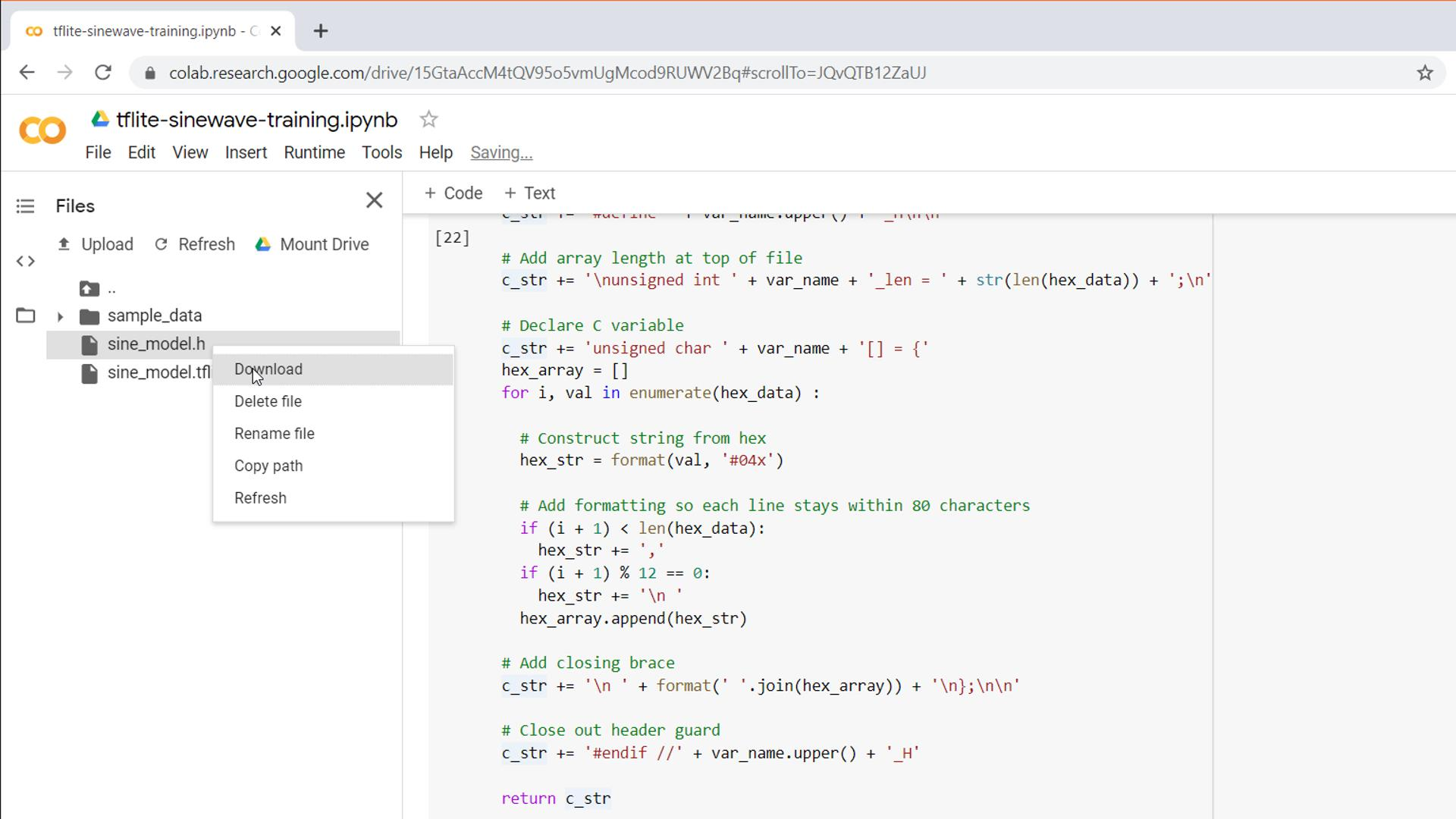You can inspect the .h file to see that it contains legitimate C code (an array length and array of lots of hex values).You can also download the sine_model.tflite model file. If you do, I recommend installing Netron to visualize the model. Simply open the .tflite file with Netron, and you should see a model description diagram. If you click on a layer, you can get information about that layer, such as the input and output types and tensor sizes.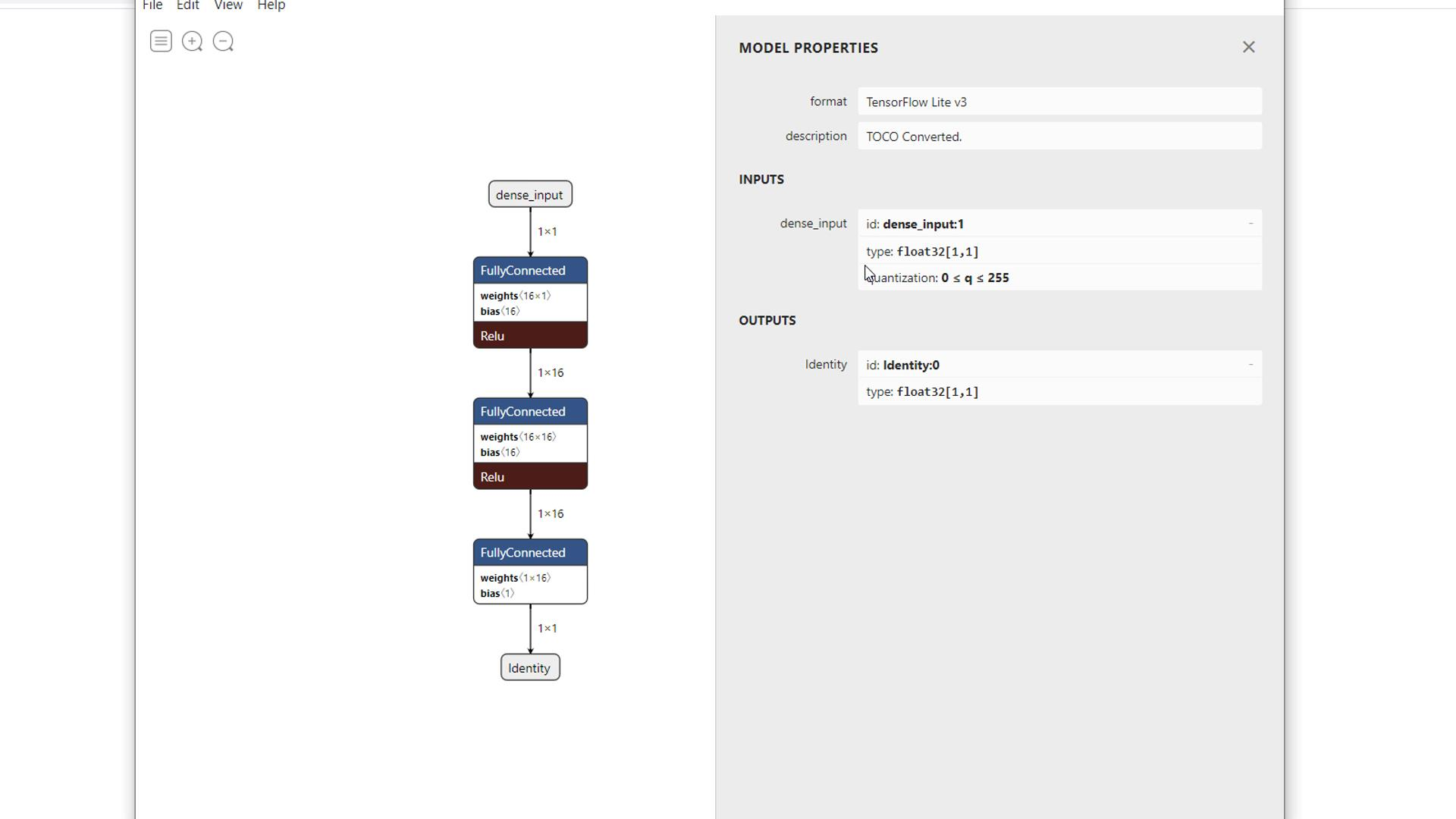Going Further

Hopefully, this has helped you get started using Colab to create a simple TensorFlow Lite model with the intention of deploying it to a microcontroller! On the next tutorial, we will run the TensorFlow Lite inference engine on an Arduino and use our model to predict sine function values.

Pete Warden’s original code can be found here: https://github.com/tensorflow/tensorflow/blob/master/tensorflow/lite/micro/examples/hello_world/create_sine_model.ipynb